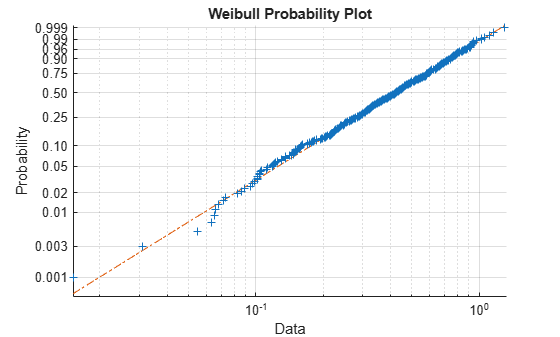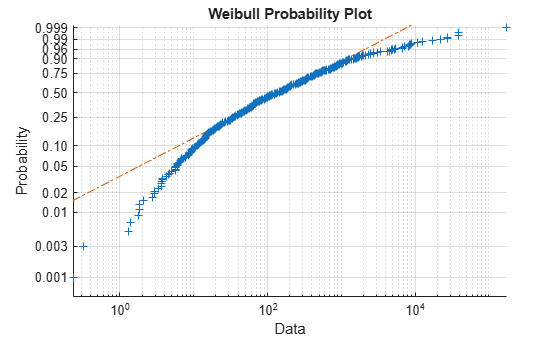# lillietest

Lilliefors test

## Syntax

``h = lillietest(x)``
``h = lillietest(x,Name,Value)``
``````[h,p] = lillietest(___)``````
``````[h,p,kstat,critval] = lillietest(___)``````

## Description

example

````h = lillietest(x)` returns a test decision for the null hypothesis that the data in vector `x` comes from a distribution in the normal family, against the alternative that it does not come from such a distribution, using a Lilliefors test. The result `h` is `1` if the test rejects the null hypothesis at the 5% significance level, and `0` otherwise.```

example

````h = lillietest(x,Name,Value)` returns a test decision with additional options specified by one or more name-value pair arguments. For example, you can test the data against a different distribution family, change the significance level, or calculate the p-value using a Monte Carlo approximation.```

example

``````[h,p] = lillietest(___)``` also returns the p-value `p`, using any of the input arguments from the previous syntaxes. ```

example

``````[h,p,kstat,critval] = lillietest(___)``` also returns the test statistic `kstat` and the critical value `critval` for the test.```

## Examples

collapse all

Load the sample data. Test the null hypothesis that car mileage, in miles per gallon (`MPG`), follows a normal distribution across different makes of cars.

```load carbig [h,p,k,c] = lillietest(MPG)```
```Warning: P is less than the smallest tabulated value, returning 0.001. ```
```h = 1 ```
```p = 1.0000e-03 ```
```k = 0.0789 ```
```c = 0.0451 ```

The test statistic `k` is greater than the critical value `c`, so `lillietest` returns a result of `h = 1` to indicate rejection of the null hypothesis at the default 5% significance level. The warning indicates that the returned $p$-value is less the smallest value in the table of precomputed values. To find a more accurate $p$-value, use `MCTol` to run a Monte Carlo approximation. See Determine the p-value Using Monte Carlo Approximation.

Load the sample data. Create a vector containing the first column of the students’ exam grades data.

```load examgrades x = grades(:,1);```

Test the null hypothesis that the sample data comes from a normal distribution at the 1% significance level.

`[h,p] = lillietest(x,'Alpha',0.01)`
```h = 0 ```
```p = 0.0348 ```

The returned value of `h = 0` indicates that `lillietest` does not reject the null hypothesis at the 1% significance level.

Load the sample data. Test the null hypothesis that car mileage, in miles per gallon (`MPG`), follows an exponential distribution across different makes of cars.

```load carbig h = lillietest(MPG,'Distribution','exponential')```
```h = 1 ```

The returned value of `h = 1` indicates that `lillietest` rejects the null hypothesis at the default 5% significance level.

Generate two sample data sets, one from a Weibull distribution and another from a lognormal distribution. Perform the Lilliefors test to assess whether each data set is from a Weibull distribution. Confirm the test decision by performing a visual comparison using a Weibull probability plot (`wblplot`).

Generate samples from a Weibull distribution.

```rng('default') data1 = wblrnd(0.5,2,[500,1]);```

Perform the Lilliefors test by using the `lillietest`. To test data for a Weibull distribution, test if the logarithm of the data has an extreme value distribution.

`h1 = lillietest(log(data1),'Distribution','extreme value')`
```h1 = 0 ```

The returned value of `h1 = 0` indicates that `lillietest` fails to reject the null hypothesis at the default 5% significance level. Confirm the test decision using a Weibull probability plot.

`wblplot(data1)`The plot indicates that the data follows a Weibull distribution.

Generate samples from a lognormal distribution.

`data2 =lognrnd(5,2,[500,1]);`

Perform the Lilliefors test.

`h2 = lillietest(log(data2),'Distribution','extreme value')`
```h2 = 1 ```

The returned value of `h2 = 1` indicates that `lillietest` rejects the null hypothesis at the default 5% significance level. Confirm the test decision using a Weibull probability plot.

`wblplot(data2)`The plot indicates that the data does not follow a Weibull distribution.

Load the sample data. Test the null hypothesis that car mileage, in miles per gallon (`MPG`), follows a normal distribution across different makes of cars. Determine the $p$-value using a Monte Carlo approximation with a maximum Monte Carlo standard error of `1e-4`.

```load carbig [h,p] = lillietest(MPG,'MCTol',1e-4)```
```h = 1 ```
```p = 8.3333e-06 ```

The returned value of `h = 1` indicates that `lillietest` rejects the null hypothesis that the data comes from a normal distribution at the 5% significance level.

## Input Arguments

collapse all

Sample data, specified as a vector.

Data Types: `single` | `double`

### Name-Value Pair Arguments

Specify optional comma-separated pairs of `Name,Value` arguments. `Name` is the argument name and `Value` is the corresponding value. `Name` must appear inside quotes. You can specify several name and value pair arguments in any order as `Name1,Value1,...,NameN,ValueN`.

Example: `'Distribution','exponential','Alpha',0.01` tests the null hypothesis that the population distribution belongs to the exponential distribution family at the 1% significance level.

Significance level of the hypothesis test, specified as the comma-separated pair consisting of `'Alpha'` and a scalar value in the range (0,1).

• If `MCTol` is not used, `Alpha` must be in the range [0.001,0.50].

• If `MCTol` is used, `Alpha` must be in the range (0,1).

Example: `'Alpha',0.01`

Data Types: `single` | `double`

Distribution family for the hypothesis test, specified as the comma-separated pair consisting of `'Distr'` and one of the following.

 `'normal'` Normal distribution `'exponential'` Exponential distribution `'extreme value'` Extreme value distribution

• To test `x` for a lognormal distribution, test if `log(x)` has a normal distribution.

• To test `x` for a Weibull distribution, test if `log(x)` has an extreme value distribution.

Example: `'Distribution','exponential'`

Maximum Monte Carlo standard error for `p`, the p-value of the test, specified as the comma-separated pair consisting of `'MCTol'` and a scalar value in the range (0,1).

Example: `'MCTol',0.001`

Data Types: `single` | `double`

## Output Arguments

collapse all

Hypothesis test result, returned as `1` or `0`.

• If `h` `= 1`, this indicates the rejection of the null hypothesis at the `Alpha` significance level.

• If `h` `= 0`, this indicates a failure to reject the null hypothesis at the `Alpha` significance level.

p-value of the test, returned as a scalar value in the range (0,1). `p` is the probability of observing a test statistic as extreme as, or more extreme than, the observed value under the null hypothesis. Small values of `p` cast doubt on the validity of the null hypothesis.

• If `MCTol` is not used, `p` is computed using inverse interpolation into the table of critical values, and is returned as a scalar value in the range [0.001,0.50]. `lillietest` warns when `p` is not found within the tabulated range and returns either the smallest or largest tabulated value.

• If `MCTol` is used, `lillietest` conducts a Monte Carlo simulation to compute a more accurate p-value, and `p` is returned as a scalar value in the range (0,1).

Test statistic, returned as a nonnegative scalar value.

Critical value for the hypothesis test, returned as a nonnegative scalar value.

collapse all

### Lilliefors Test

The Lilliefors test is a two-sided goodness-of-fit test suitable when the parameters of the null distribution are unknown and must be estimated. This is in contrast to the one-sample Kolmogorov-Smirnov test, which requires the null distribution to be completely specified.

The Lilliefors test statistic is:

`${D}^{*}=\underset{x}{\mathrm{max}}|\stackrel{^}{F}\left(x\right)-G\left(x\right)|,$`

where $\stackrel{^}{F}\left(x\right)$ is the empirical cdf of the sample data and $G\left(x\right)$ is the cdf of the hypothesized distribution with estimated parameters equal to the sample parameters.

`lillietest` can be used to test whether the data vector `x` has a lognormal or Weibull distribution by applying a transformation to the data vector and running the appropriate Lilliefors test:

• To test `x` for a lognormal distribution, test if `log(x)` has a normal distribution.

• To test `x` for a Weibull distribution, test if `log(x)` has an extreme value distribution.

The Lilliefors test cannot be used when the null hypothesis is not a location-scale family of distributions.

### Monte Carlo Standard Error

The Monte Carlo standard error is the error due to simulating the p-value.

The Monte Carlo standard error is calculated as:

`$SE=\sqrt{\frac{\left(\stackrel{^}{p}\right)\left(1-\stackrel{^}{p}\right)}{\text{mcreps}}},$`

where $\stackrel{^}{p}$ is the estimated p-value of the hypothesis test, and `mcreps` is the number of Monte Carlo replications performed.

The number of Monte Carlo replications, `mcreps`, is determined such that the Monte Carlo standard error for $\stackrel{^}{p}$ less than the value specified for `MCTol`.

## Algorithms

To compute the critical value for the hypothesis test, `lillietest` interpolates into a table of critical values pre-computed using Monte Carlo simulation for sample sizes less than 1000 and significance levels between 0.001 and 0.50. The table used by `lillietest` is larger and more accurate than the table originally introduced by Lilliefors. If a more accurate p-value is desired, or if the desired significance level is less than 0.001 or greater than 0.50, the `MCTol` input argument can be used to run a Monte Carlo simulation to calculate the p-value more exactly.

When the computed value of the test statistic is greater than the critical value, `lillietest` rejects the null hypothesis at significance level `Alpha`.

`lillietest` treats `NaN` values in `x` as missing values and ignores them.

 Conover, W. J. Practical Nonparametric Statistics. Hoboken, NJ: John Wiley & Sons, Inc., 1980.

 Lilliefors, H. W. “On the Kolmogorov-Smirnov test for the exponential distribution with mean unknown.” Journal of the American Statistical Association. Vol. 64, 1969, pp. 387–389.

 Lilliefors, H. W. “On the Kolmogorov-Smirnov test for normality with mean and variance unknown.” Journal of the American Statistical Association. Vol. 62, 1967, pp. 399–402.